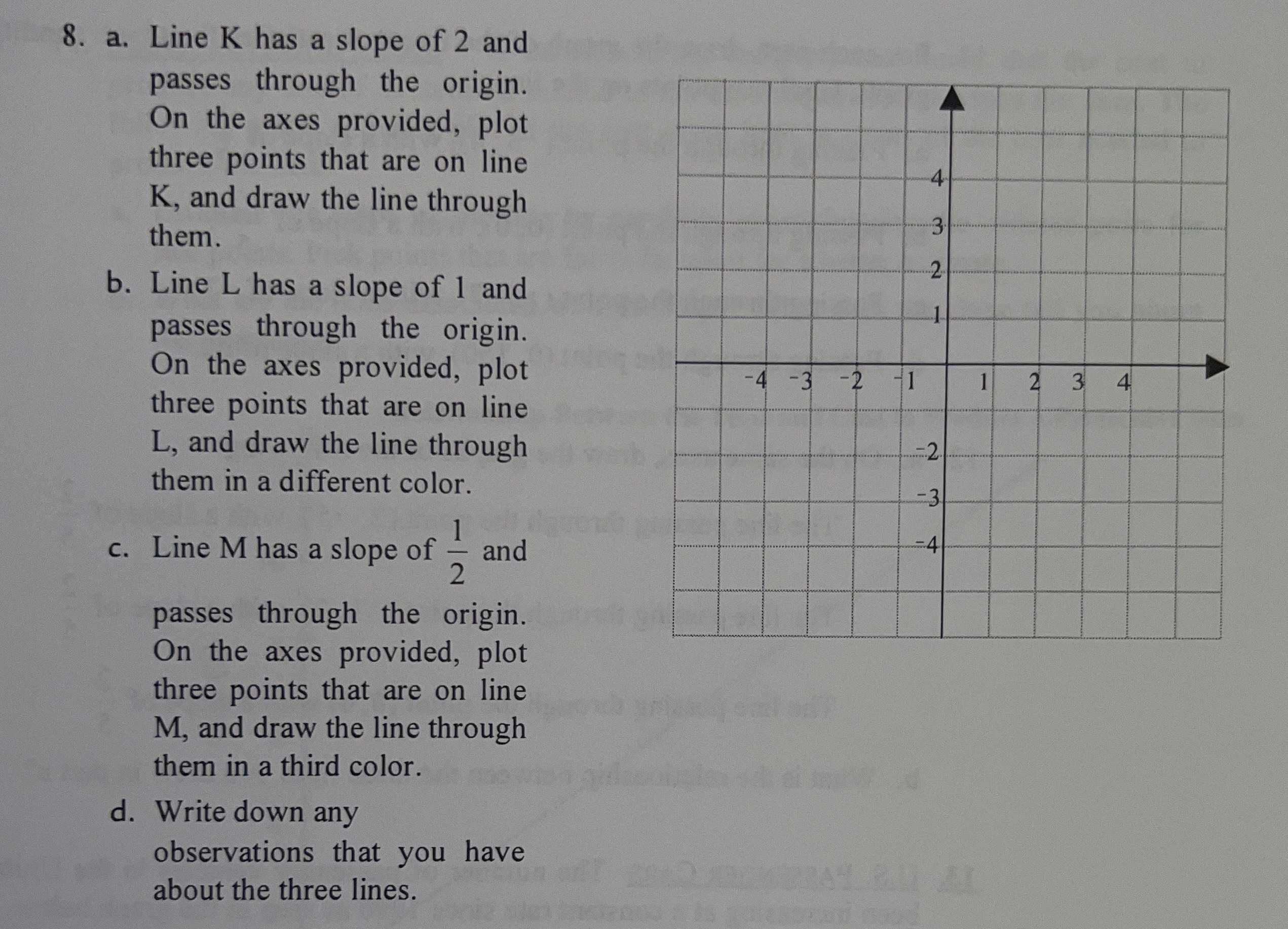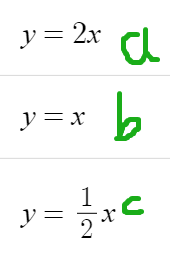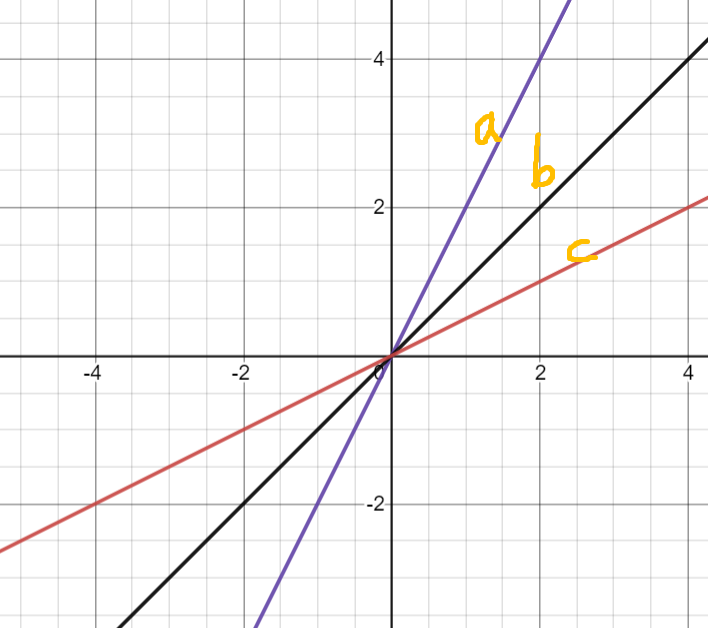### ¿Todavía tienes preguntas de matemáticas?

Pregunte a nuestros tutores expertos
Algebra
Pregunta8. a. Line $$K$$ has a slope of $$2$$ and passes through the origin. On the axes provided, plot three points that are on line K, and draw the line through them. b. Line $$L$$ has a slope of 1 and passes through the origin. On the axes provided, plot three points that are on line L, and draw the line through them in a different color. c. Line M has a slope of $$\frac { 1 } { 2 }$$ and passes through the origin. On the axes provided, plot three points that are on line M, and draw the line through them in a third color. d. Write down any observations that you have about the three lines.